# Understanding the Value of Uniswap v3 Liquidity Positions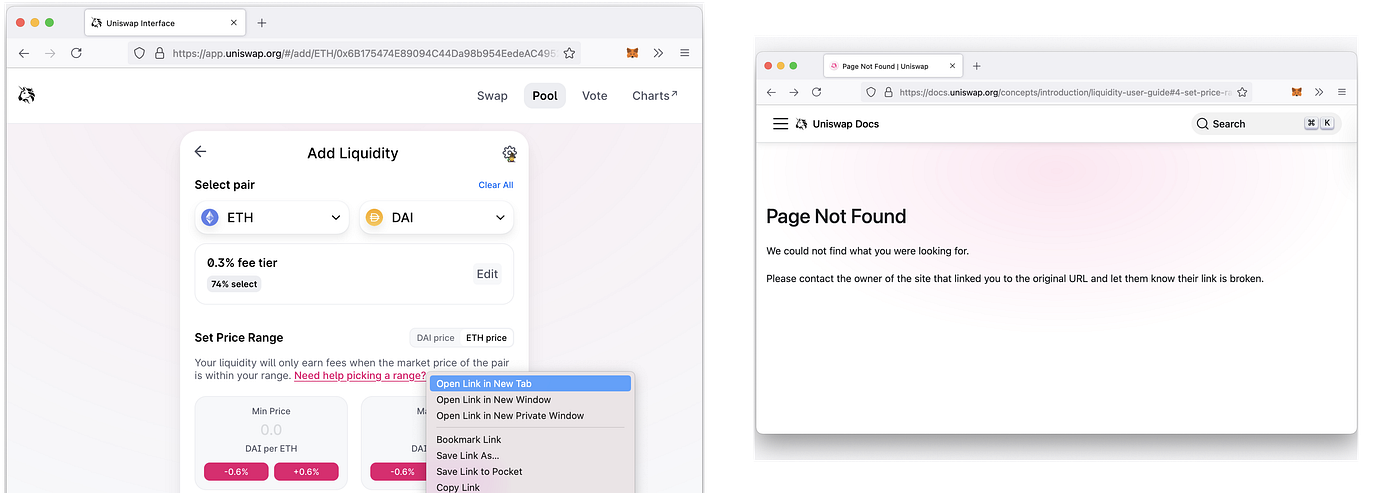Looking for guidance in picking a Uniswap v3 liquidity range. Seeking help leads nowhere.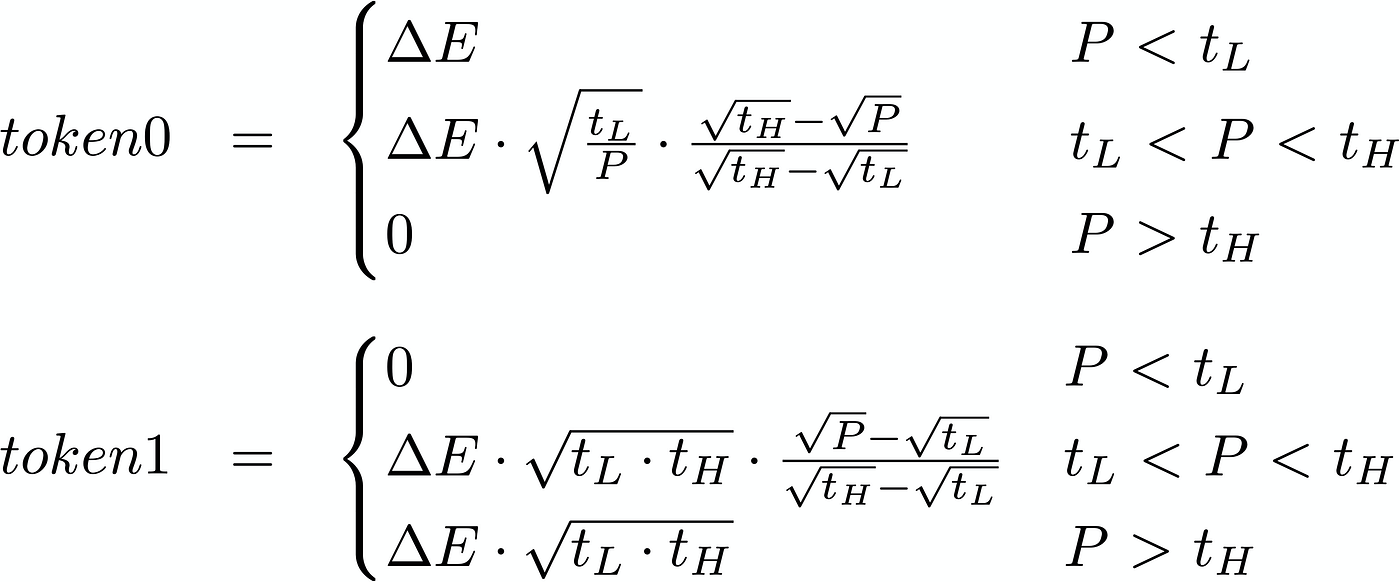Token composition of a LP position. Describes how much of token0 and token1 are in a LP position.

# Computing Delta, the rate of change in Net Liq ValueDelta of a LP position. How much does the value of a LP position changes when the price of the underlying asset changes by \$1?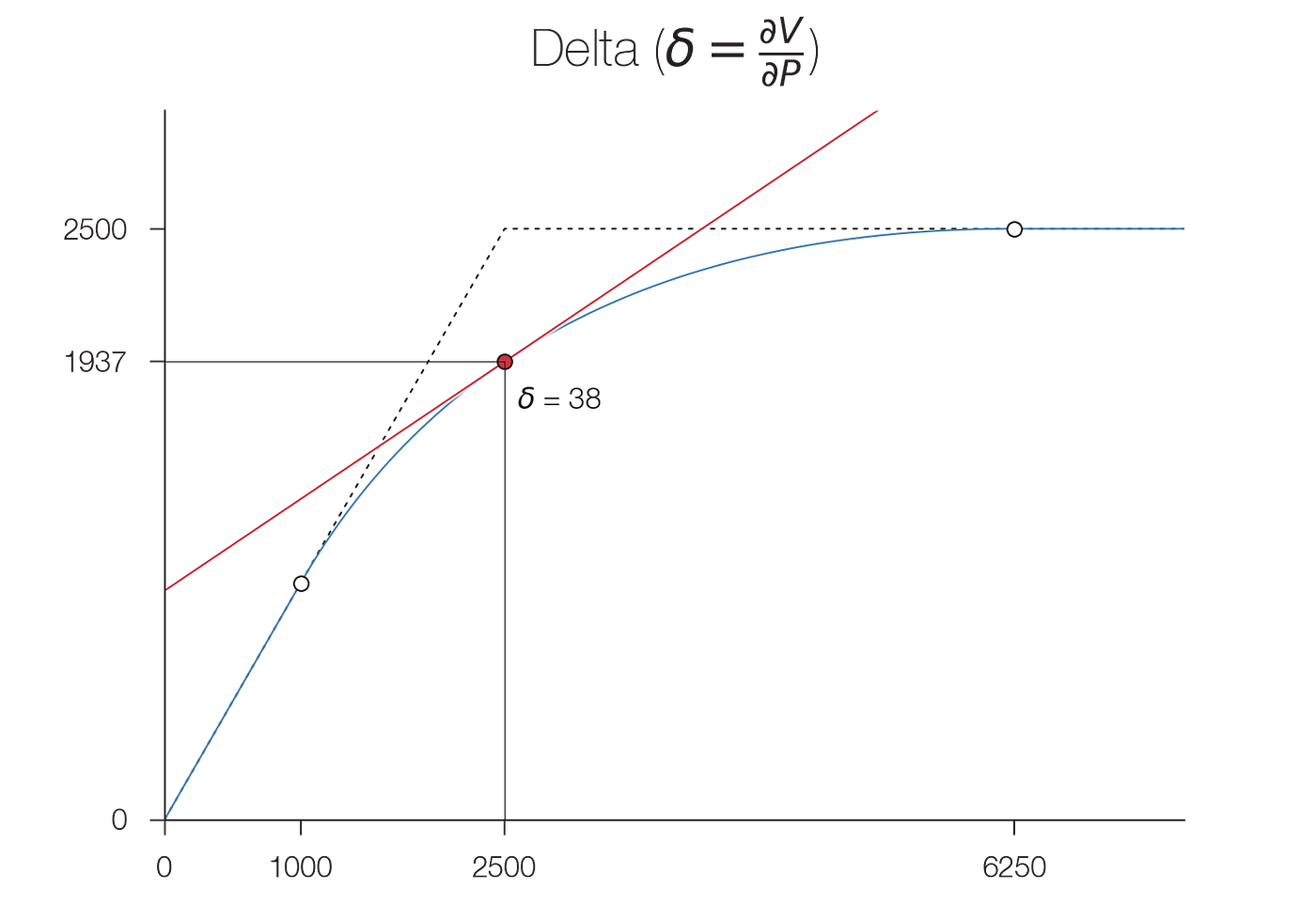Delta as a slope. The slope of the red curve represents the value of delta for the normalized LP position 1*sqrtV(P). The slope changes from 100% to 0% as the price changes between the lower tick tL and the upper tick tH. Download GIF version.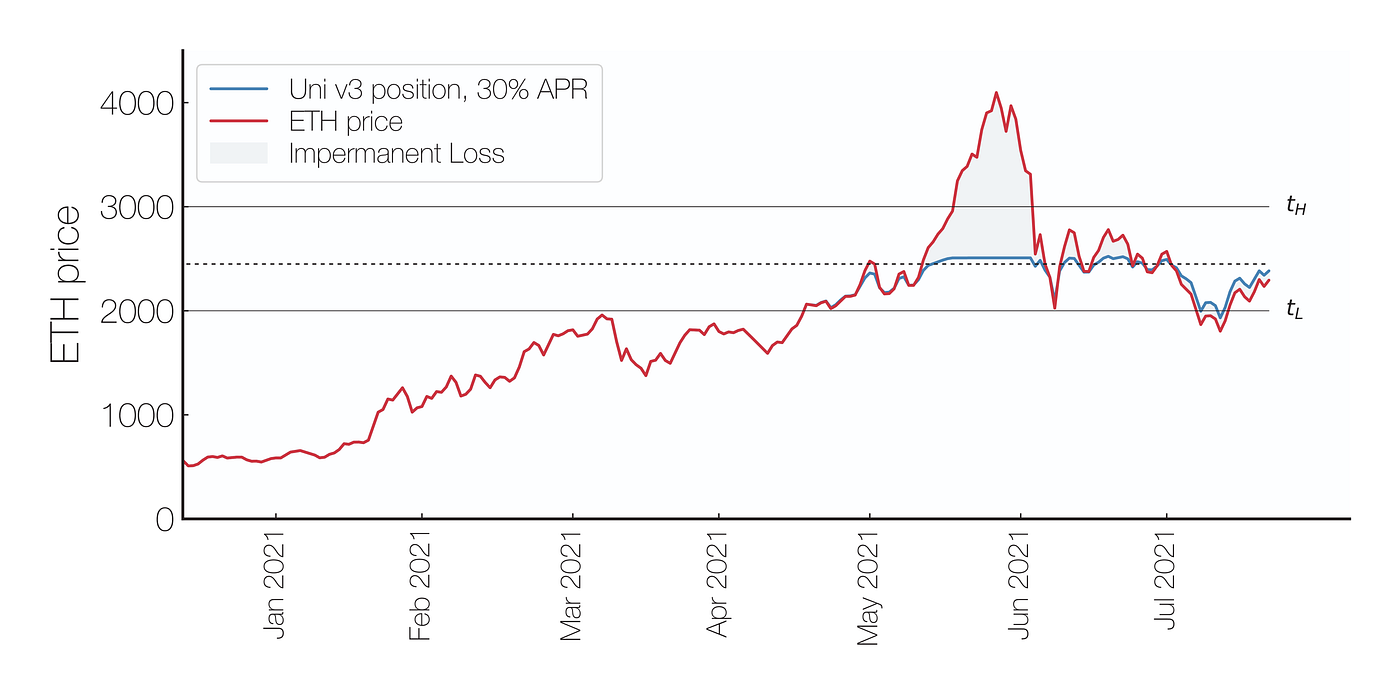Ranged Covered Call. Return of a LP position defined by an ETH price between 2000 and 3000. The LP position’s value will track the price of ETH below the lower tick tL and will remain unchanged when the price of ETH is above the upper tick 3000. Returns from fees are approximately 8%.

# Understanding the impact of Net Delta on returns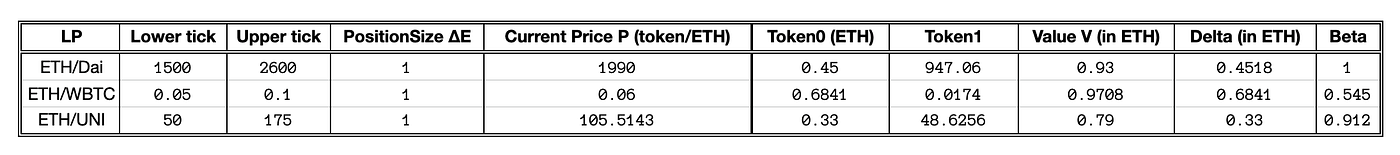LP portfolio. Details of a portfolio consisting of ETH/Dai, ETH/WBTC and ETH/UNI LP positions. The total value and the delta have been converted into ETH to simplify the net delta calculation.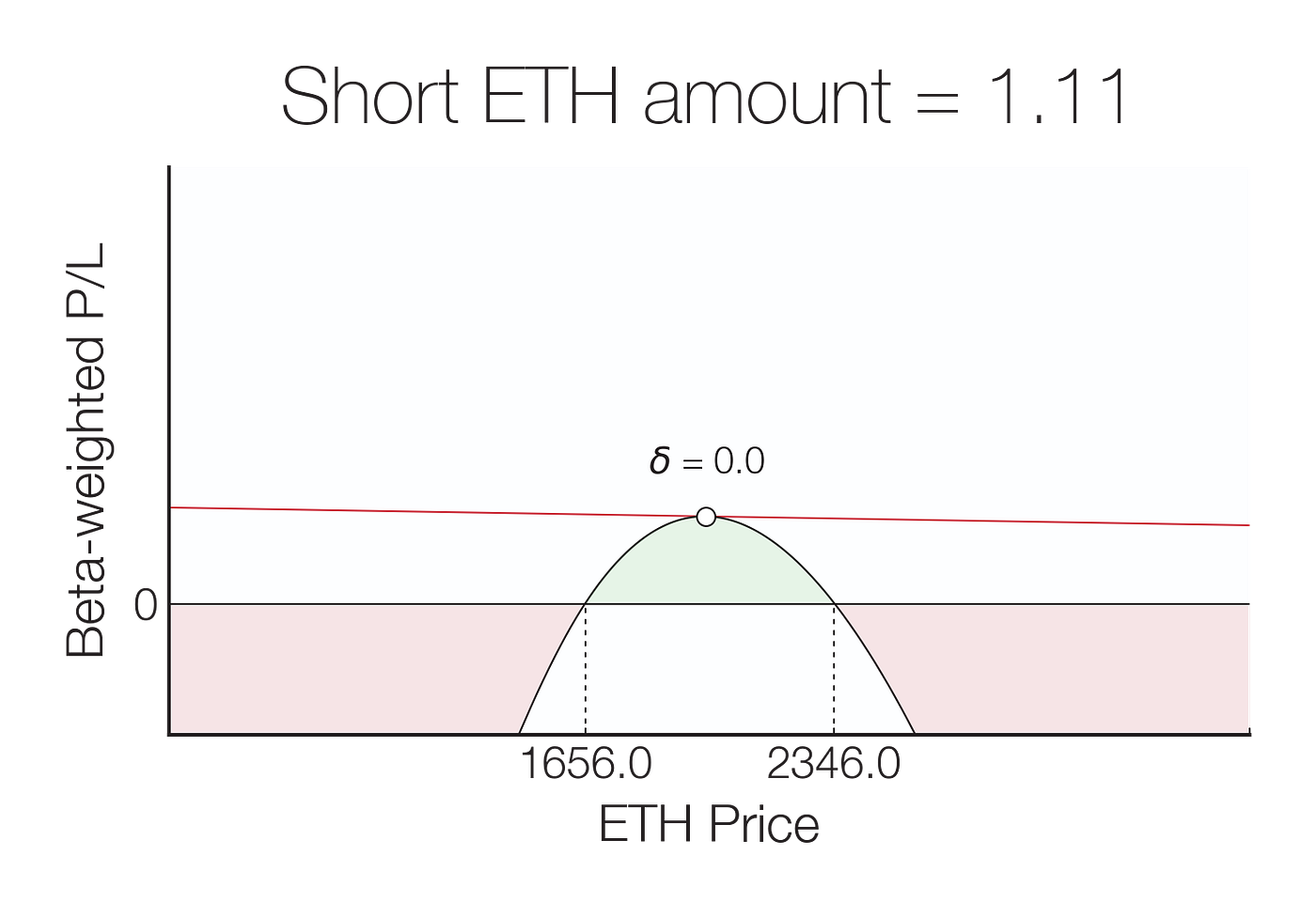Net delta of a ETH/Dai, ETH/WBTC, and ETH/UNI portfolio. The beta-weighted expected return of the portfolio will change according to the amount of ETH shorted to balancer the delta of the position. Tuning the amount of shorted ETH shifts the position’s break-even points. We assumed an initial 100 Dai in fees were collected to compute the beta-weighted P/L for this position. Download GIF version.

--

--